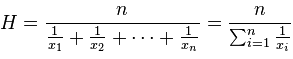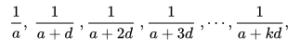# Harmonic Mean / Progression: Definition, Examples

Harmonic mean: Contents:

## What is the Harmonic Mean?

The harmonic mean is a very specific type of average. It’s generally used when dealing with averages of units, like speed or other rates and ratios.

The formula is:If the formula above looks daunting, all you need to do to solve it is:

• Add the reciprocals of the numbers in the set.
• Divide the number of items in the set by your answer to Step 1.

Not that great with calculating reciprocals? Try this online calculator.

## Examples

Watch the video or read on below for a few examples:

What is the harmonic mean of 1, 5, 8, 10?

• Add the reciprocals of the numbers in the set: 1/1 + 1/5 + 1/8 + 1/10 = 1.425
• Divide the number of items in the set by your answer to Step 1. There are 4 items in the set, so:
4 / 1.425 = 2.80702

Tip: Check your calculations with this online calculator.

## Harmonic mean in statistics

The harmonic mean is used in some very specific use cases in statistics. For example, it is used in population genetics when calculating the effects of fluctuations in the census population size (i.e., the entire population) on the effective population size (i.e., the number of individuals effectively contributing to the population’s gene pool).

The harmonic mean for random samples is calculated with the above formula.

## Difference Between the Harmonic Mean and Arithmetic Mean

The difference between the two means is a little bit of a mind-bender, so if you don’t quite get the concept at first, you aren’t alone. I can remember having to read the explanation several times and doing some examples on paper to assure myself it was correct.

Here is an example of a problem involving (the more common) arithmetic mean:

• Joe drives a car at 20 mph for the first hour and 30 mph for the second. What’s his average speed?
• The average speed is 20 + 30 / 2 = 25 mph.

And now, the same example with the harmonic mean:

• Joe drives a car at 20 mph for the half of the journey and 30 mph for the second half. What’s his average speed?
• For this problem note that we’re being told he went a certain speed for a journey segment. We need the harmonic mean:
• = 2/(1/20 + 1/30)
• = 2(0.05 + 0.033)
• = 2 / 0.083
• = 24.09624 mph.

The difference between these two is that the first problem is calculating an average speed based on time, while the second is based on distance. You’ll notice that the harmonic mean is slightly less than the arithmetic mean. This is always the case, that the harmonic mean will be the lowest average.

## Harmonic Progression

A harmonic progression is a sequence of numbers where each term is the harmonic mean of the neighboring terms. A “progression” is just a sequence of numbers that follows a pattern.

A harmonic progression takes the form:In this formula:

## Alternate Definition of harmonic mean

You can also make this sequence with reciprocals of an arithmetic progression (an arithmetic progression is a series of numbers where each successive term has a constant added to it). More specifically, if the following sequence is an arithmetic progression:Then it is also a harmonic progression, as long as the numbers are non-zero.

## Properties of the Harmonic Progression

A harmonic progression is a sequence of numbers where the harmonic mean of any two consecutive terms is the same.

One interesting property of the harmonic progression is that, unless a = 1 and k = 0, it can never sum up to an integer. The reason for this is that at least one of the denominators of the progression will be divisible by a prime number that doesn’t divide any of the other denominators.

## Examples of Harmonic Progressions

Let’s say a and d are both ½. Then the harmonic progression will equal:
12, 6, 4, 3, 12/5, 2…n.
The nth term will always be 12/(1 + n).

Suppose a was 1/10 and d was – 2/30. Then the terms of the progression would equal:
10, 30, -30, -10, -6, -30/7…n.
The nth term will always be 10/(1 – 2n/3).

The graph below shows part of the harmonic progression where an = 1 / n. For this series, a and d are both 1. The first term, 1/n, is 1, the second term is 1/(1 + 1) =2, and so on.Sometimes, this progression is referred to as “The” harmonic series.

## Harmonic mean: References

Joyce, D. Introduction to Series and Sequences. Math 221 Calculus. Published Spring 2013. Retrieved from https://www2.clarku.edu/faculty/djoyce/ma121/seqser.pdf on March 30, 2019.
Zenth, Tommy “The Sum of the Harmonic Progression as an Integral.” From Wolfram Demonstrations Project, Published online March 7, 2011. Retrieved from http://demonstrations.wolfram.com/TheSumOfTheHarmonicProgressionAsAnIntegral/ on March 30, 2019.

CITE THIS AS:
Stephanie Glen. "Harmonic Mean / Progression: Definition, Examples" From StatisticsHowTo.com: Elementary Statistics for the rest of us! https://www.statisticshowto.com/calculus-definitions/harmonic-mean/